# Texas Go Math Grade 1 Lesson 20.3 Answer Key Spend and Save

Refer to our Texas Go Math Grade 1 Answer Key Pdf to score good marks in the exams. Test yourself by practicing the problems from Texas Go Math Grade 1 Lesson 20.3 Answer Key Spend and Save.

## Texas Go Math Grade 1 Lesson 20.3 Answer Key Spend and Save

Explore

Which items might you need for breakfast? Which items do you not need?1, 4, 5 , 6 items are need for breakfast and item 2 is not need for breakfast.

FOR THE TEACHER • Suppose you are grocery shopping for items you need for breakfast. Circle the items you might need. Cross out the items you do not need. Draw one more item you might need.

Math Talk
Mathematical Processes

How do you decided what food you need to buy for breakfast?

Model and Draw

Jon earns 100 each week for three weeks. He can save the money or spend the money.
Save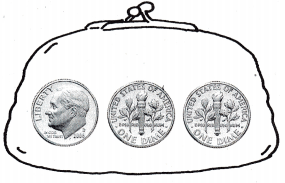10¢ ______ ______
Jon has 30¢.
What does save mean?
Given that
Jon earn for each week = 100
Jon earns for 3 weeks = 100 × 3 = 300
Jon left 30¢
So, Jon spends the money.
Spend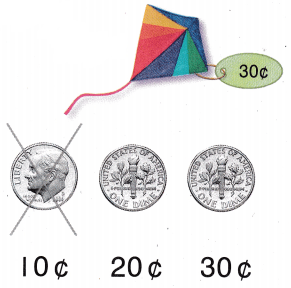Jon has 0¢
What does spend mean?

Share and Show

Use coins. Draw and label the coins you use. Write the answer.

Question 1.
Erica saves 120 each week for four weeks. How much money does Erica save?
Erica saves 120 for each week.
For four weeks she saves = 120 × 4 = 480¢.
Erica save = 480¢.

Question 2.
David has 15¢. He spends 8¢ to buy a toy airplane. How much money does David have left?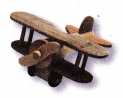Given that
David has 15¢
He spends on 8¢ to buy a toy aeroplane.
How much money David left = 15¢ – 8¢ = 7¢.

Problem Solving

Use coins. Draw and label the coins you use. Write the answer.

Question 3.
Sam saves 15¢ each week for three weeks. How much money does Sam save?
Given that
Sam saves 15¢ for each week.
For three weeks Sam save = 3 × 15 = 45¢
Total money Sam save = 45¢.

Question 4.
Jodi has 16¢. She spends 7¢ to buy a gift for her sister. How much money does Jodi have left?Given that
Jodi have left = 16¢ – 7¢ = 9¢

Question 5.

H.O.T. Multi-Step Maya saves 20¢ on Monday, 40¢ on Tuesday, and 10¢ on Wednesday. How much money does Maya save?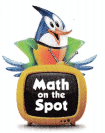Given that
Maya saves on Monday = 30¢
Maya saves on Tuesday = 40¢
Maya saves on Wednesday = 10¢
Maya saves = 30¢ + 40¢ + 10¢ = 80¢.

Choose the correct answer. Draw to show what you did to solve the problem. You may use coins.

Question 6.
Amy spends 20¢ each week for three weeks. How much money does Amy spend?
(A) 40¢
(B) 60¢
(C) 23¢
Given that
Amy spends 20¢ for each week.
Amy spends for three weeks = 20¢ × 3 = 60¢
Option B is the correct answer.

Question 7.
Apply Reid saves 11¢ each week for 6 weeks. How much money does Reid save?
(A) 61¢
(B) 17¢
(C) 66¢
Reid saves 11¢ for each week.
Reid save for 6 weeks = 6 × 11 = 66¢
Total money Reid save = 66¢
Option C is the correct answer

Question 8.
Brian has 12¢. He spends 7¢ to buy a pencil. How much money does Brian have left?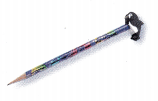(A) 5¢
(B) 19¢
(C) 10¢
Given that
Brian has 12¢
He spends 7¢ to buy a pencil.
Brian left money = 12¢ – 7¢ = 5¢
Option A is the correct answer.

Question 9.
TEXAS Test Prep Chris has 50¢. He puts 3 dimes in his bank, and uses the rest to buy a baseball. How much does Chris spend on the baseball?
(A) 30¢
(B) 80¢
(C) 20¢
Given that
Chris has 50¢.

He puts 3 dimes in the bank.

1 dime = 10 cents then 3 dimes = 30 cents.

And he use the rest to by a baseball.

Chris spend on the baseball = 50¢ – 30 ¢ = 20 ¢.

### Texas Go Math Grade 1 Lesson 20.3 Homework and Practice Answer Key

Use coins. Draw and label the coins you use. Write the answer.

Question 1.
Devon saves 11¢ each week for five weeks. How much money does Devon save?
Given that
Devon saves 11¢ each week.
For Five weeks Devon save = 5 × 11¢ = 55¢
Therefore Devon save = 55¢.

Problem Solving

Use coins. Draw and label the coins you use. Write the answer.

Question 2.
Maxi has 18¢. She spends 9¢ to buy a horn for her bike. How much money does Maxi have left?Given that
Maxi has 18¢
She spends 9¢ to buy a bike horn.
Maxi left money = 18¢ – 9¢ = 9¢
Therefore total money left near Maxi = 9¢.

Question 3.

Multi-Step Brian saves 50¢ on Monday and 30¢ on Tuesday. Then he spends 20¢ to rent a movie. How much money does Brian have left?Given that
Brian saves on Monday = 50¢
Brian saves on Tuesday = 20¢
Total money Brian saves = 50¢ + 20¢ = 70¢.
Then he spends 20¢ on the movie.
Brian left money = 50¢

Lesson Check

Choose the correct answer. You may use coins.

Question 4.
Nan spends 10¢ each day for five days. How much money does Nan spend?
(A) 25¢
(B) 50¢
(C) 15¢
Given that
Nan spends 10¢ for each day
For five days he spends = 5 × 10¢ = 50¢.
Option B is the correct answer.

Question 5
Ric saves 12e each week for 3 weeks. How much money does Ric save?

(A) 36¢
(B) 33¢
(C) 15¢
Ric saves each week = 12¢
Ric saves for 3 weeks = 12¢ × 3¢ = 36¢
Option A is the correct answer.

Question 6.
Abby has 16¢. She spends 9¢ to buy a card. How much money does Abby have left?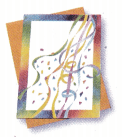(A) 7¢
(B) 9¢
(C) 25¢
Given that
Abby has 16¢
She spends 9¢ for buy a cards
Abby had left money = 16¢ – 9¢ = 7¢
Total money he left = 7¢.
Option A is the correct answer.

Question 7.
Maria has 70¢. She spends 20¢ for hair ribbons and saves the rest. How much money does Maria save?(A) 95¢
(B) 45¢
(C) 50¢